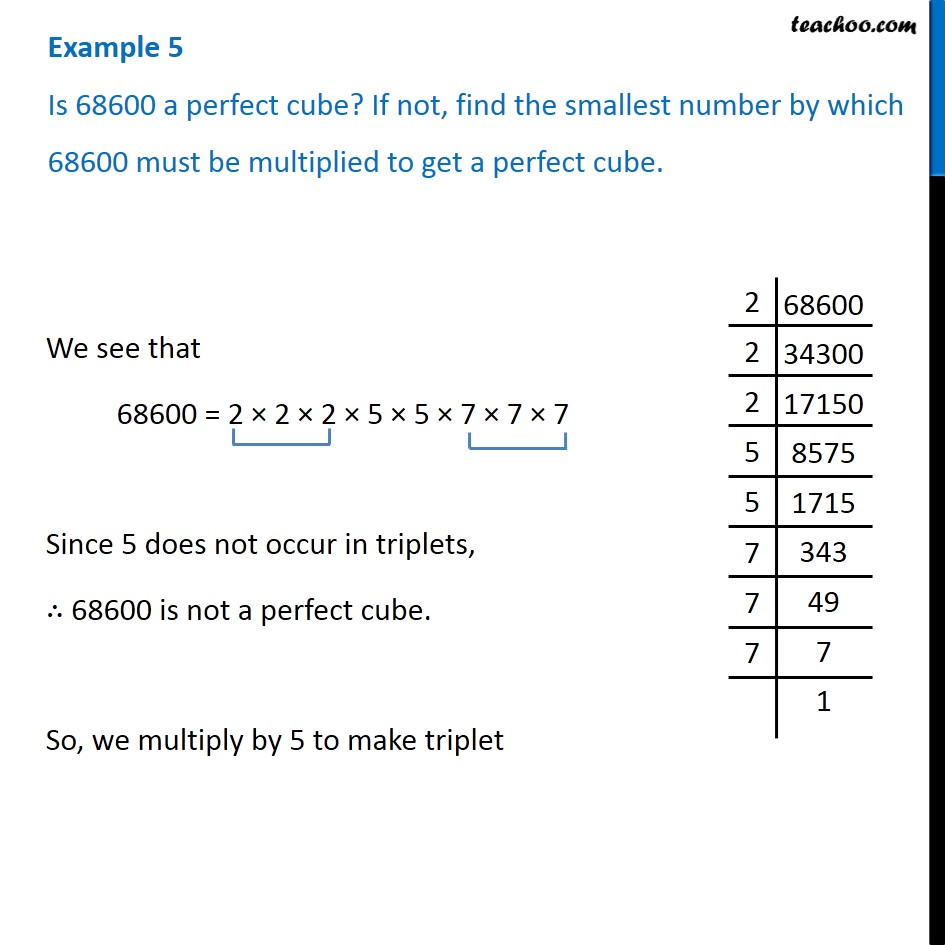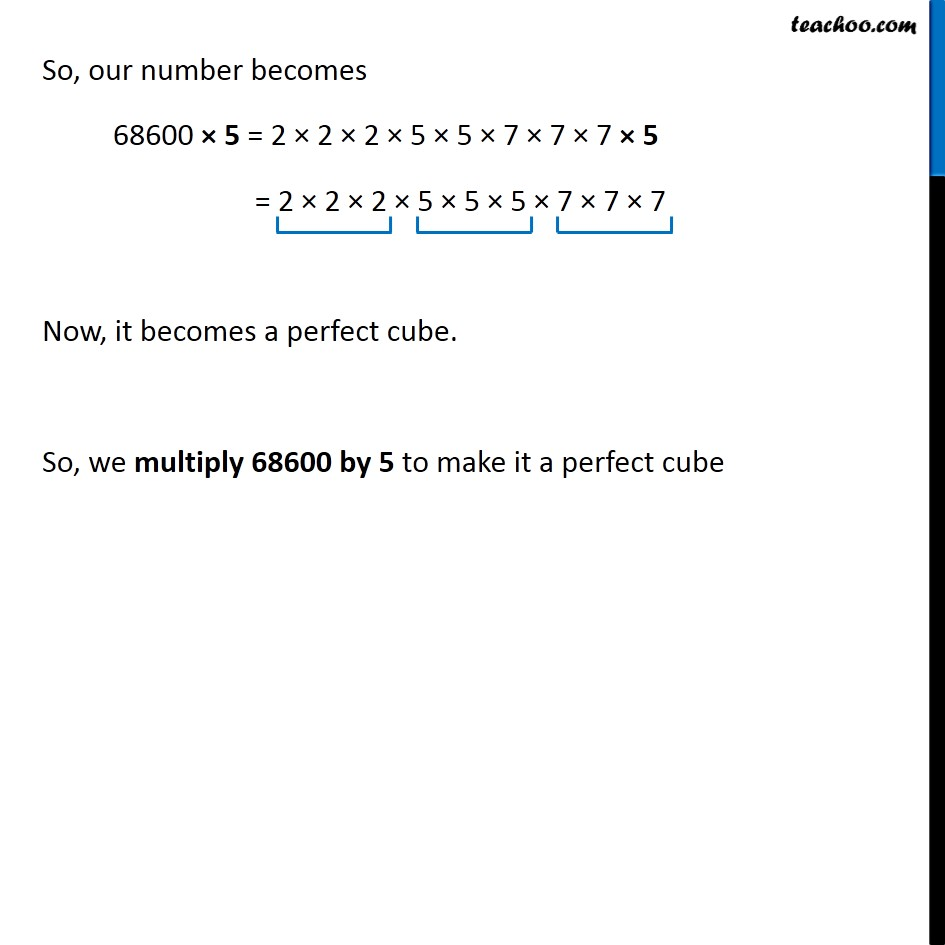Subscribe to our Youtube Channel - https://you.tube/teachoo

1. Chapter 7 Class 8 Cubes and Cube Roots
2. Concept wise
3. Smallest number multiplied to get a perfect cube

Transcript

Example 5 Is 68600 a perfect cube? If not, find the smallest number by which 68600 must be multiplied to get a perfect cube. We see that 68600 = 2 × 2 × 2 × 5 × 5 × 7 × 7 × 7 Since 5 does not occur in triplets, ∴ 68600 is not a perfect cube. So, we multiply by 5 to make triplet So, our number becomes 68600 × 5 = 2 × 2 × 2 × 5 × 5 × 7 × 7 × 7 × 5 = 2 × 2 × 2 × 5 × 5 × 5 × 7 × 7 × 7 Now, it becomes a perfect cube. So, we multiply 68600 by 5 to make it a perfect cube

Smallest number multiplied to get a perfect cube